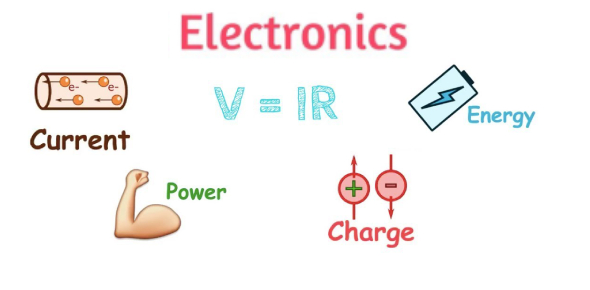# Electronics Basics! Trivia Knowledge! Quiz

14 Questions | Attempts: 358
ShareSettings• 1.
What is the definition of impedance?
• A.

A Combination Of Resistance And Reactance, Expressed In Ohms

• B.

Dc Resistance Of A Speaker Voice Coil

• C.

All Of These Choices

• D.

The Square Root Of The Voice Coil Resistance

• 2.
What is the value of a resistor that has the following color bands: red, red, red, gold?
• A.

220 Ohms, 10%

• B.

2.2k Ohms, 5%

• C.

2k Ohms, 10%

• D.

22 Ohms, 5%

• 3.
What is the SI unit for potential difference?
• A.

Current

• B.

Volt

• C.

Ampere

• D.

Watt

• 4.
Two resistors are wired in parallel. Which of the following statements is true?
• A.

The Total Resistance Will Be Less Than The Sum Of The Two

• B.

The Total Resistance Will Be The Difference Between The Two Resistors

• C.

The Total Resistance Will Be The Sum Of Both Resistors

• D.

The Total Resistance Will Be Greater Than The Sum Of The Two

• 5.
Name the three leads of a field-effect transistor (FET)?
• A.

Source, Gate, And Drain

• B.

Emitter, Collector, And Base

• C.

Cathode, Plate, And Anode

• D.

Source, Gate, And Base

• 6.
What is an inductor called that is designed for the specific purpose of passing DC while blocking AC?
• A.

Rectifier Diode

• B.

Triac

• C.

Choke

• D.

None Of These Choices

• 7.
A capacitor is best described by which one of the following statements?
• A.

A Device That Store Electrical Energy And Blocks The Flow Of DC

• B.

A Single Electrode Device That Amplifies Current

• C.

A Component That Is Used To Provide A Definite Amount Of Resistance

• D.

A Device That Stores Electrical Energy And Blocks The Flow Of AC

• 8.
The electron flow in a circuit passes through a NPN transistor from _________ to ___________?
• A.

Emitter, Collector

• B.

Emitter, Base

• C.

Base, Emitter

• D.

Collector, Base

• 9.
What is the main difference between the function of a SCR and a triac?
• A.

The Scr Is A Switching Device, While The Triac Is An Amplifying Device

• B.

The Scr Is Made If Silicon And The Triac Is Not

• C.

All Of These Choices

• D.

The Scr Can Be Triggered In Only One Direction, While The Triac Can Be Switched On In Either Direction

• 10.
What is the distinguishing feature of a thyristor?
• A.

A Momentary Pulse Of Gate Current Switches Them On

• B.

They Are Less Expensive Than Transistors

• C.

They Switch Faster Than Transistors

• D.

They Are The Only High Power Semiconductors Available

• 11.
In a basic transistor circuit, what is the most significant factor in determining if a transistor performs as a switch or as an amplifier?
• A.

All Of These Choices

• B.

The Transistor Package Style

• C.

The Control Circuit

• D.

Transistor Type

• 12.
Why are PNP transistors used less often than NPN transistors?
• A.

Pnps Switch Slower And Are Generally More Expensive Than Npns

• B.

Pnps Are Only Used In Digital Circuits

• C.

Pnps Are Newer, So They Have To Catch Up

• D.

The Function Of A Pnp Isn't As Useful As A Npn

• 13.
A P-channel enhancement type MOSFET performs much the same function as a PNP transistor. What is the main difference?
• A.

A Mosfet Switches Much Faster

• B.

A Mosfet Is Considerably Larger

• C.

A Mosfet Is Controlled By Voltage Rather Than Current

• D.

A Mosfet Regulates Current, But Is Non-Switching

• 14.
Why is a transistor defined as a an amplifying device?
• A.

All Of These Choices

• B.

The Current In The Output Circuit Is Greater Than The Current In The Input Circuit

• C.

Because The Collector Collects Electrons

• D.

The Voltage At The Emitter Is Greater Than The Voltage At The Base

## Related TopicsBack to top
×

Wait!
Here's an interesting quiz for you.